# A short introduction to the spathial Package

The spathial package is a generic tool for manifold analysis. It allows to infer a relevant transition or evolutionary path which can highlights the features involved in a specific process. spathial can be useful in all the scenarios where the temporal (or pseudo-temporal) evolution is the main problem (e.g. tumor progression).

The spathial package implements the principal path algorithm  and allows to navigate a n-dimensional space. The input of the algorithm are two points, which represent the boundary conditions of the algorithm: the starting and the ending points. Given the boundaries, the algorithm learns a smooth transition path connecting them. Along the path there are new intermediate data samples which gradually morphs from the starting point to the ending point. In this way it is possible to move from two known states. Additionally, analysing the correlation between the path progression and the features is possible to perform feature selection. The workflow for constructing the path is composed of few steps. Firstly, it is necessary to choose the starting and the ending points. spathial provides three different alternatives to do that (which will be discussed later), but the user can additionally choose his own strategy. The second step consist in prefiltering the data. This step is non strictly necessary to construct the path, but allows to obtain a local solution removing some of the data points. In this way, the solution is based on a restricted number of samples. Finally, it is possible to run the principal path algorithm.

spathial additionally provides functions to analize the path. In particular it allows to plot data in 2d using the t-SNE algorithm  for dimensionality reduction, to learn the labels corresponding to each path’s intermediate points using the kNN algorithm  and to compute the Pearson’s correlation (and the associated p_value and q_value) of the features with respect to the path progression. The latter allows to find the features involved in the transition process from the start point to the end point.

The next sections describes how to use spathial and provides some examples.

# Quick start

In this section the most basic steps to run the spathial implementation of the principal path algorithm  are shown, through a simple 2d example.

The very first step is the spathial package installation

install.packages("spathial")

library("spathial")

To compute the principal path one assumes to have an input matrix X. Each column of the matrix X is a feature (e.g. a gene) and each row has a univocal name (e.g. a sample). For a supervised solution, one also assumes that the input vector X_labels is present which contains for each row of X a description label (e.g. the sample category). Otherwise, the unsupervised solution can be obtained with X_labels = NULL. For the sake of simplicity, a simple .csv file with 900 samples and 3 columns (2 + labels) is provided. The following code snippet shows how to load the .csv and how to properly format the data.

# Load the dataset with 900 samples
myfile<-system.file("extdata", "2D_constellation.csv", package = "spathial")
head(data)
##    feature1  feature2 label
## 1  0.440620 -0.206480    c8
## 2 -0.305250  0.122770    c3
## 3 -0.234850  0.097566    c8
## 4  0.205200 -0.058423    c8
## 5  0.022774  0.134450    c8
## 6 -0.223260  0.092053    c8
# Vector X_labels
X_labels <- data$label X_labels_num <- data$numLabels
# Data Matrix X
X <- data[,2:(ncol(data)-2)]
rownames(X)<-paste0("sam",rownames(X))

This is how the data matrix X should look like at this point

head(X)
##       feature2  feature1
## sam1 -0.206480  0.440620
## sam2  0.122770 -0.305250
## sam3  0.097566 -0.234850
## sam4 -0.058423  0.205200
## sam5  0.134450  0.022774
## sam6  0.092053 -0.223260

The sample labels vector X_labels, assigns categories to each sample, coherently with rows order in X

head(X_labels)
##  "c8" "c3" "c8" "c8" "c8" "c8"

The following code snippet shows how to plot the data points colored according to X_labels:

# Plot the results
colors <- rainbow(length(table(as.numeric(as.factor(X_labels)))))
colors_labels <- sapply(as.numeric(as.factor(X_labels)), function(x){colors[x]})
par(mar=c(5.1, 4.1, 4.1, 8.1), xpd=TRUE)
plot(X[,1],X[,2],col=colors_labels,pch=as.character(as.numeric(as.factor(X_labels))),
xlab=colnames(X),ylab=colnames(X), main="Data points")
legend_names = unique(X_labels)
legend_color = unique(colors_labels)
legend_pch = unique(as.character(as.numeric(as.factor(X_labels))))
legend("topright", inset=c(-0.25,0), legend=legend_names, col=legend_color, pch=legend_pch)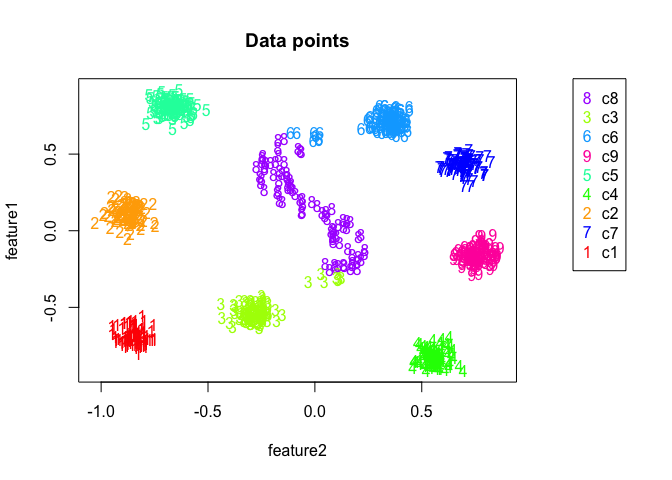Quick Start example dataset

par(oldpar)

## Step 1: select the boundaries

The first step to compute the principal path consists in selecting the starting and the ending points. The package spathial has the function spathialBoundaryIds which takes as input the matrix X, the corresponding labels X_labels (or NULL) and the parameters mode, from and to.

The parameter mode can assume of one the following values:

• 1 (default): the user can choose the starting and the ending points directly from the 2D representations of the data points. In this case the values of the parameters from and to are not considered.
• 2: the starting point is the centroid of all the data points labelled as the parameter from, while the ending point is the centroid of all the data points labelled as the parameter to. This mode is allowed only when X_labels is not NULL.
• 3: the starting point is the data point with the univocal rowname equal to the parameter from while the ending point is the data point with the univocal rowname equal to the parameter to.

The output of the function spathialBoundaryIds is a list with the following content:

• X: the initial input matrix X plus the starting and the ending points;
• X_labels: the initial vector X_labels inclusive of the labels of the starting and the ending points;
• boundary_ids: the rowname of the starting and the ending points.

N.B. The matrix X and the vector X_labels change only when mode=2 (the resulting matrix and vector have two additional elements, corresponding to the centroids).

User can choose the starting and the ending points with their own strategy, which can be more suitable for their specific problem; in this case, the user should run the function spathialBoundaryIds with mode=3 specifing the rownames of the samples using the parameters from and to.

The following code chunks show how to use the function spathialBoundaryIds, with different values of the parameter mode, and how to extract the output:

# mode=1 (User-selected)
boundary_init <- spathial::spathialBoundaryIds(X, X_labels, mode=1)
# mode=2 (From named label centroid to another label centroid)
boundary_init <- spathial::spathialBoundaryIds(X, X_labels, mode=2, from="c3", to="c6")
# mode=3 (From named sample to another named sample)
boundary_init <- spathial::spathialBoundaryIds(X, X_labels, mode=3,
from="sample123", to="sample456")

Once the boundaries are defined, and only in the case of mode=2, the X and X_labels objects inside the boundary_init object contain extra meta-samples (the boundaries) and thus the original X and X_labels need to be updated accordingly:

# Take the output from the variable boundary_init
boundary_ids<-boundary_init$boundary_ids X<-boundary_init$X
X_labels<-boundary_init$X_labels The following plots the boundaries when mode=2, from=3 and to=6: #Plot the results boundaries <- X[which(rownames(X) == boundary_ids | rownames(X) == boundary_ids),] oldpar <- par(no.readonly = TRUE) par(mar=c(5.1, 4.1, 4.1, 8.1), xpd=TRUE) plot(X[,1],X[,2], col=colors_labels, pch=as.character(as.numeric(as.factor(X_labels))), xlab=colnames(X), ylab=colnames(X), main="Boundary points") points(boundaries[,1],boundaries[,2], pch="x",col="black",cex=4) legend_names = c(unique(X_labels), "boundaries") legend_color = c(unique(colors_labels), "black") legend_pch = c(unique(as.character(as.numeric(as.factor(X_labels)))), "X") legend("topright", inset=c(-0.3,0), legend=legend_names, col=legend_color, pch=legend_pch)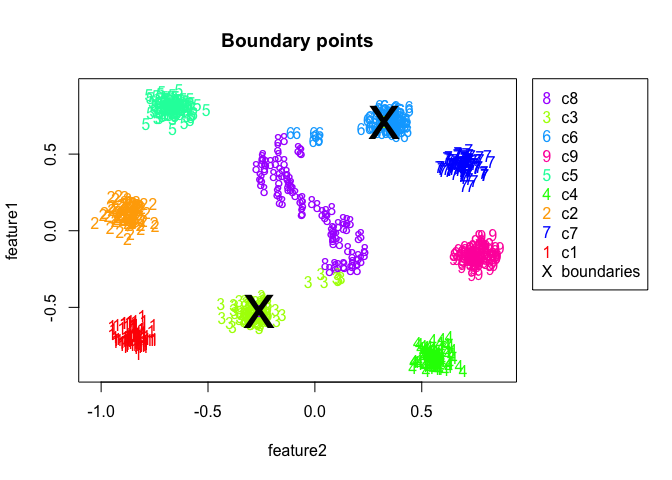Quick Start example - boundaries par(oldpar) ## Step 3: prefiltering (optional) The principal path algorithm is intrinsically global. If one is searching for a path which does not involve the whole dataset, one can run the function spathialPrefiltering before the principal path algorithm is applied. If one wants to run spathial on the entire dataset, this filtering procedure is not due. The function spathialPrefiltering takes as input the matrix X and the boundaries boundary_ids which are the result of the function spathialBoundaryIds. The output of the function spathialPrefiltering is a list with the following content: • mask: the indexes of the samples to preserve; • boundary_ids_filtered: the rowname of the starting and the ending points. The following code shows how to use the function spathialPrefiltering and how to extract the output. The function remove some samples and they are stored in the X_garbage matrix. The mask vector contains the samples to be kept. # Prefilter data filter_res <- spathial::spathialPrefiltering(X, boundary_ids) mask <- filter_res$mask
boundary_ids <- filter_res$boundary_ids # Plot the results boundaries <- X[which(rownames(X) == boundary_ids | rownames(X) == boundary_ids),] X_garbage <- X[!mask,] The following code shows the results of the prefiltering step, with removed points greyed out: oldpar <- par(no.readonly = TRUE) par(mfrow=c(2,1)) par(mar=c(5.1, 4.1, 4.1, 8.1), xpd=TRUE) plot(X[,1],X[,2], col=colors_labels, pch=as.character(as.numeric(as.factor(X_labels))), xlab=colnames(X), ylab=colnames(X), main="Before Filtering") points(boundaries[,1],boundaries[,2], pch="x",col="black",cex=4) legend_names = c(unique(X_labels), "boundaries") legend_color = c(unique(colors_labels), "black") legend_pch = c(unique(as.character(as.numeric(as.factor(X_labels)))), "X") legend("topright", inset=c(-0.3,0), legend=legend_names, col=legend_color, pch=legend_pch) par(mar=c(5.1, 4.1, 4.1, 8.1), xpd=TRUE) plot(X[,1],X[,2], col=colors_labels, pch=as.character(as.numeric(as.factor(X_labels))), main="After Filtering", xlab=colnames(X),ylab=colnames(X)) points(boundaries[,1],boundaries[,2], pch="x",col="black",cex=4) points(X_garbage[,1],X_garbage[,2], col="gray", pch=4) legend_names = c(unique(X_labels), "boundaries", "filtered") legend_color = c(unique(colors_labels), "black", "gray") legend_pch = c(unique(as.character(as.numeric(as.factor(X_labels)))), "X", "x") legend("topright", inset=c(-0.3,0), legend=legend_names, col=legend_color, pch=legend_pch)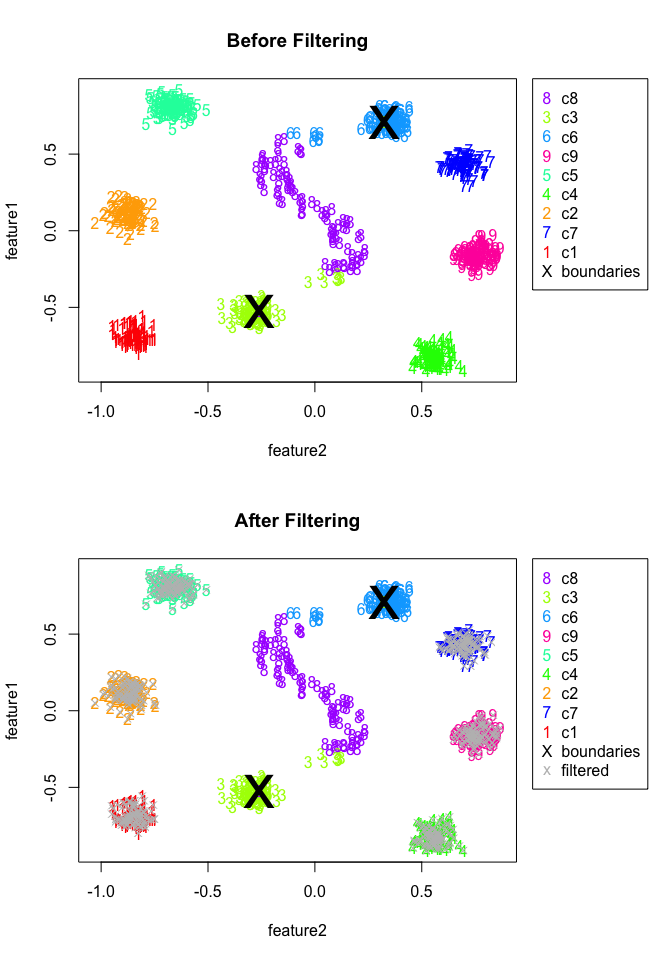Quick Start example - prefiltering par(oldpar) ## Step 4: principal path The function spathialWay executes the principal path algorithm and gives as output the waypoints. It takes as input the matrix X, the boundaries boundary_ids and the parameter NC, which is the desired number of waypoints of the resulting principal path. For example, given NC=10 the resulting path will be composed of 10 waypoints plus the starting and the ending points. The output of the function spathialWay is the variable spathial_res, which contains the coordinates of the waypoints of the principal path; The following code shows how to use the function spathialWay, with or without prefiltering, and how to get the output: # Compute principal path without prefiltering NC <- 50 spathial_res_without_filtering <- spathial::spathialWay(X, boundary_ids, NC) # Compute principal path after prefiltering X_filtered <- X[mask,] X_labels_filtered <- X_labels[mask] NC <- 50 spathial_res_with_filtering <- spathial::spathialWay(X_filtered, boundary_ids, NC) The next subsection shows how to get results from the output object spathial_res. ## Step 5: understanding the results The package spathial includes three different functions to interpret the output of the principal path algorithm: spathialPlot, spathialLables and spathialStatistics. The following subsections describe what they do and how to use each of them. ### Plotting the principal path The function spathialPlot plots the principal path together with all the data points (either filtered or not filtered) together with the boundaries. This function takes as input the matrix X (the initial version), the vector X_labels (or NULL), the boundaries boundary_ids, the output of the principal path algorithm spathial_res, the parameter perplexity value (default 30), the parameter mask which is one of the results of the prefiltering and it is NULL when the prefiltering is not computed and the parameter title which is the title of the plot and can be NULL. When the input matrix X has more than 2 columns, the function reduce the dimension of the space from N (>2) to 2 using the t-SNE algorithm . The following code shows how to use the function spathialPlot with the path generated during the previous step (with or without prefiltering): # Plot principal path with prefiltering - provide a mask spathial::spathialPlot(X, X_labels, boundary_ids, spathial_res_with_filtering, perplexity_value=30, mask=mask, title="Principal path with prefiltering" )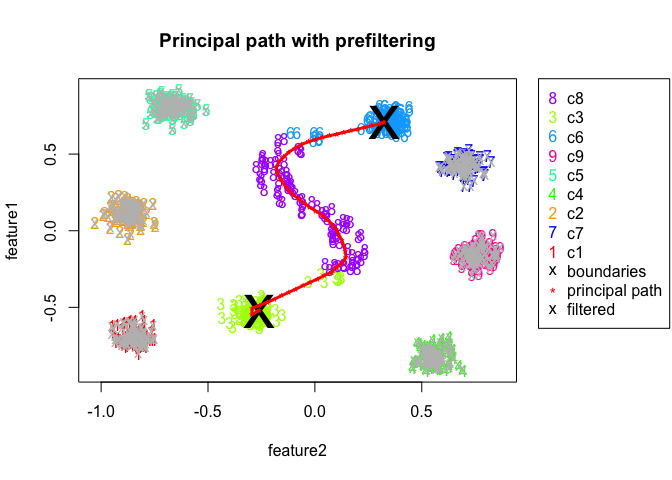Output of the spathialPlot() function. # Plot principal path without prefiltering - mask NULL spathial::spathialPlot(X, X_labels, boundary_ids, spathial_res_without_filtering, perplexity_value=30, title="Principal path without prefiltering" )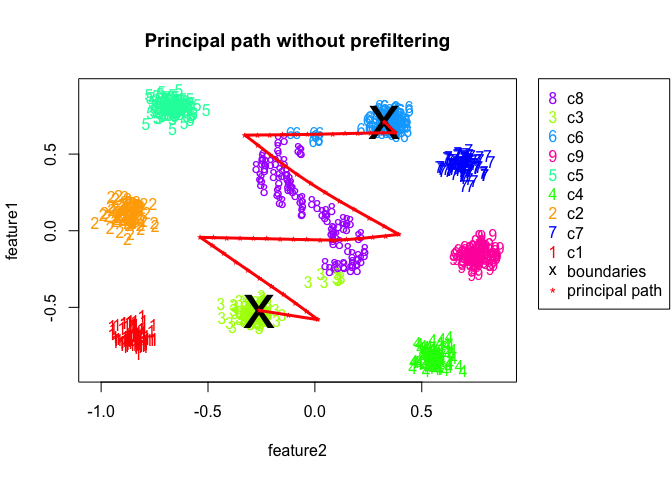### Assessing the path progression The function spathialLabels predicts the label for each waypoint of the principal path by detecting the nearest sample . This function is available only when X_labels != NULL and could be particularly useful when one wants to find the breakpoints between one class and the other. The function takes as input the matrix X (which is composed only by the preserved samples if the prefiltering was used), the vector X_labels and the output object of the principal path algorithm spathial_res. The output are the labels for each waypoint of the principal path. The following code shows how to use the function spathialLabels and how to plot the result: ### Matrix X not filtered ppath_labels <- spathial::spathialLabels(X, X_labels, spathial_res_with_filtering) # Plot the results ppath_labels_numerical = as.numeric(as.factor(ppath_labels)) colors_labels_ppath <- sapply(ppath_labels_numerical, function(y){colors[as.integer(y)]}) plot(c(1:length(ppath_labels_numerical)), c(ppath_labels_numerical), col=colors_labels_ppath, pch=as.character(ppath_labels_numerical), xlab="Path Step", ylab="Sample Label", main="Path progression with prefiltering" )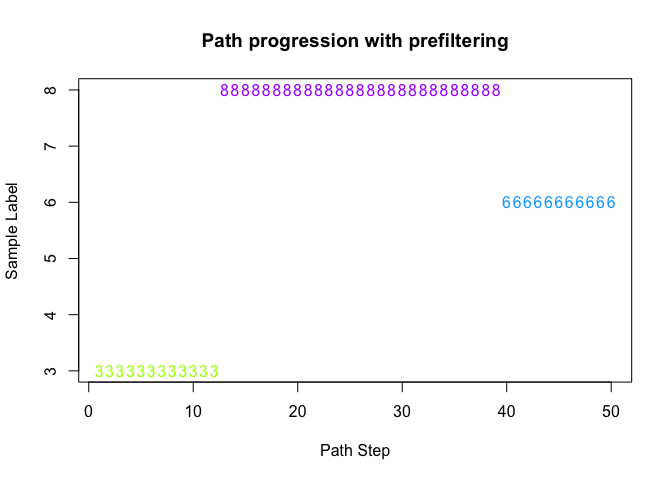Quick Start example - path labels across path steps ### Matrix X filtered ppath_labels <- spathial::spathialLabels(X, X_labels, spathial_res_without_filtering) # Plot the results ppath_labels_numerical = as.numeric(as.factor(ppath_labels)) colors_labels_ppath <- sapply(ppath_labels_numerical, function(y){colors[as.integer(y)]}) plot(c(1:length(ppath_labels_numerical)), c(ppath_labels_numerical), col=colors_labels_ppath, pch=as.character(ppath_labels_numerical), xlab="Path Step", ylab="Sample Label", main="Path progression without prefiltering" )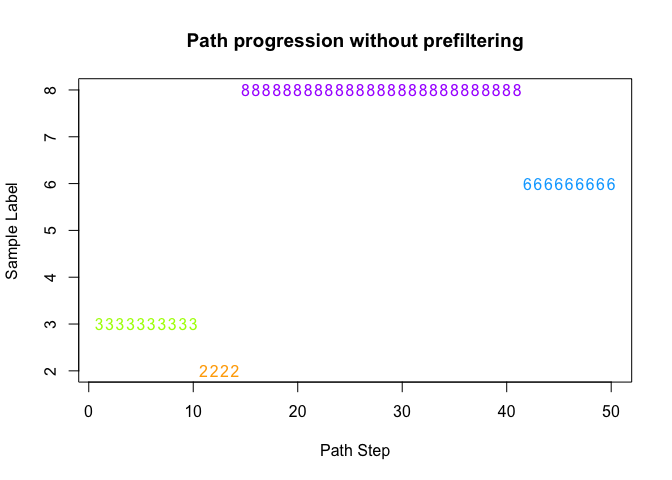Quick Start example - path labels across path steps ### Extract principal path associated features The function spathialStatistics returns statistics for each feature, based on their relation with the principal path calculated and stored in spathial_res. In particular, here one wants to understand how much each feature (a coordinate of the N-dimensional space) is correlated with the evolution of the principal path, that is to detect evolutive features. This is a path-centric way to perform feature selection. For this reason, the output of the function spathialStatistics is a list that attaches to features a progression score. • correlations: This vector contains the Pearson’s correlation coefficients between each feature and the path. • rank_scores: This vector contains the ranks of associations between the n features and the path (1 being the most positively correlated, and n the most negatively correlated). • p_value: This vector contains the p values from the Pearson’s correlation scores. • p_adj: This vector containes the p values adjusted according to the Benjamini & Hochberg (BH) method. The following code shows how to use the function spathialStatistics and to extract the correlation-based association between features and the path. # Calculate Association Statistics for each feature in the path statistics <- spathial::spathialStatistics(spathial_res_with_filtering) # Extract Pearson correlation coefficients between features and path statistics$correlations
##  feature2  feature1
## 0.5134595 0.9944206
# Calculate Association Statistics for each feature in the path
statistics <- spathial::spathialStatistics(spathial_res_without_filtering)

# Extract Pearson correlation coefficients between features and path
statistics$correlations ## feature2 feature1 ## 0.5062762 0.9672803 # A slightly more complex example In this section it is shown a higher-dimensional example, with 100 features. In this case the input matrix X is a reduced version of the TCGA Liver Cancer RNA-Seq dataset which is composed only by the 100 features (gene expression profiles, RPM-normalized) with the highest variance across the dataset. The vector X_labels contains the information about the samples. In particular, the label is “Tumor” for tumor samples, and “Normal” for normal samples, collected in the same dataset. In this case, the aim of the example is to navigate the space from the centroid of the normal tissue samples (label “Normal”) to the centroid of the tumor samples (label “Tumor”) in order to gradually morphing from one histological state to the other. For this reason, one can use the function spathialBoundaries with mode=2 specifying from="Normal" and to="Tumor". The prefiltering won’t be executed since one is searching for a global solution. The following code blocks shows how to compute the principal path algorithm. First, one loads the data from a CSV file containing RPM-normalized gene expression data: # Load data myfile<-system.file("extdata", "liver_tcga_example1.csv", package = "spathial") data<-read.csv(myfile,as.is=TRUE,header=TRUE,row.names=1) data[1:4,1:5] ## ALB HP MT.ATP6 APOA2 MT.ATP8 ## TCGA-UB-A7MB-01A-11R-A33R-07 593956.4 92680.9 170568.5 691801.2 221968.2 ## TCGA-CC-A9FW-01A-11R-A37K-07 513497.3 280958.0 301123.4 598087.7 318292.9 ## TCGA-DD-AAEE-01A-11R-A41C-07 456418.2 200048.9 422911.6 1187913.9 334117.4 ## TCGA-FV-A3R3-01A-11R-A22L-07 184082.4 172949.5 1041332.0 1116072.0 757033.8 Then one transforms it into two objects: the numeric gene expression profiles (X) and the associations between samples and samples category (X_labels), where “Tumor” indicates a tumor sample, and “Normal” a normal tissue sample. X <- data[,1:(ncol(data)-1)] X_labels <- data[,"Category"] X[1:4,1:5] ## ALB HP MT.ATP6 APOA2 MT.ATP8 ## TCGA-UB-A7MB-01A-11R-A33R-07 593956.4 92680.9 170568.5 691801.2 221968.2 ## TCGA-CC-A9FW-01A-11R-A37K-07 513497.3 280958.0 301123.4 598087.7 318292.9 ## TCGA-DD-AAEE-01A-11R-A41C-07 456418.2 200048.9 422911.6 1187913.9 334117.4 ## TCGA-FV-A3R3-01A-11R-A22L-07 184082.4 172949.5 1041332.0 1116072.0 757033.8 # Choose the starting and the ending points boundary_init <- spathial::spathialBoundaryIds(X, X_labels, mode=2, from=2, to=1) # Alternative, mode 3: # from="TCGA-DD-A39W-11A-11R-A213-07", to="TCGA-G3-AAV2-01A-11R-A37K-07" boundary_ids <- boundary_init$boundary_ids
X <- boundary_init$X X_labels <- boundary_init$X_labels

# run spathial
NC <- 50
spathial_res <- spathial::spathialWay(X, boundary_ids, NC)

In order to visualize the multi-dimensional dataset on two dimensions, one performs a t-SNE analysis on it .

# Plot the path in 2D using Rtsne
spathial::spathialPlot(X, X_labels, boundary_ids, spathial_res,
perplexity_value=30)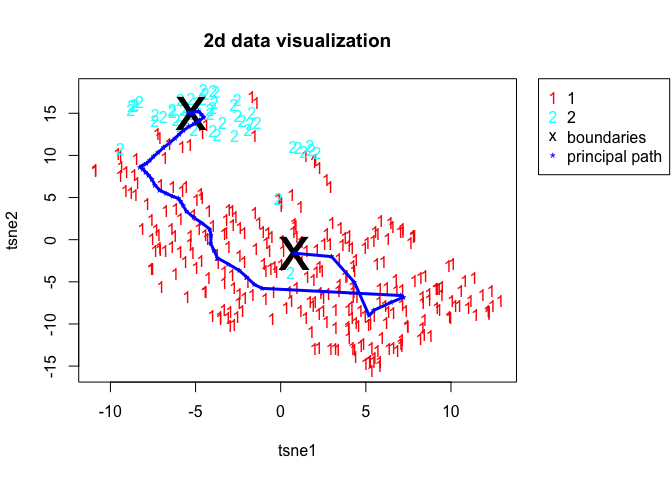Principal Path across the TCGA Liver Cancer dataset

# Labels for each waypoint with knn
ppath_labels <- spathial::spathialLabels(X, X_labels, spathial_res)
# Plot the results
colors <- rainbow(length(table(as.numeric(as.factor(X_labels)))))
ppath_labels <- as.vector(ppath_labels)
colors_labels_ppath <- sapply(ppath_labels, function(y){colors[as.integer(y)]})
plot(c(1:length(ppath_labels)), c(ppath_labels), col=colors_labels_ppath,
pch=as.character(ppath_labels), xlab="Path Step", ylab="Sample Label",
main="Path progression"
)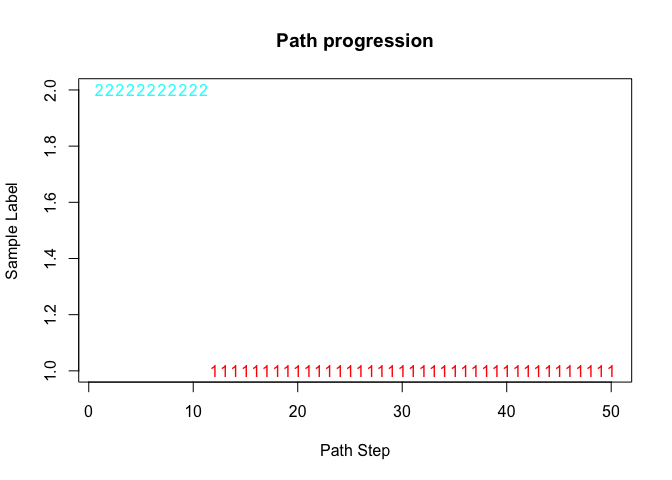Liver Cancer example - path labels across path steps

Then one can quickly extract the gene expression profiles most correlated with the path:

# Correlation along the path
statistics<-spathialStatistics(spathial_res)

In this case, the statistics is particularly interesting, as it shows the most associated functional genes to the transition between normal and tumor liver tissues. In fact, it contains information about the correlation between each feature (genes) and a vector from 1 to NC+2 (where NC is the number of waypoints) that represents the progression along the path. This helps finding the genes particularly involved with the evolution from the healthy to the unhealthy state. The following code highlights the 10 most positively and most negatively associated genes to the normal-tumor path progression:

singlepath_correlations<-statistics$correlations top_positive_correlations<-sort(singlepath_correlations,decreasing=TRUE)[1:10] top_positive_correlations ## RPS19 RPL30 RPS18 RPL13A RPS27 RPL8 RPL39 RPL10 ## 0.9489431 0.9334733 0.9334049 0.9213973 0.9158444 0.9149281 0.9145781 0.9133683 ## RPS16 RPS21 ## 0.9061229 0.9025649 top_negative_correlations<-sort(singlepath_correlations,decreasing=FALSE)[1:10] top_negative_correlations ## RBP4 ALDOB APOC3 ADH1B FGA HP FGG ## -0.9851525 -0.9655488 -0.9615431 -0.9576245 -0.9492497 -0.9374311 -0.9301608 ## TTR FGB MT2A ## -0.9298318 -0.9235418 -0.9208846 # A real case study In this section it is shown a real case study on TCGA Lung Cancer RNA-Seq dataset. In this case the input matrix X is the fully TCGA Lung Cancer RNA-Seq dataset, with 19637 features (gene expression profiles, RPM-normalized) and 562 samples. Each column of matrix X represents a feature and each row is the gene expression profile of a specific sample. The vector X_labels contains the annotations of the samples (“Tumor” or “Normal”) which can be extracted from the TCGA barcode. We provide an .rda file with both the X and X_labels. One can simply load it by computing the following command. load(url("https://github.com/erikagardini/spathial_dataframes/raw/master/luad_tcga.rda")) The aim of the example is to navigate the space from the normal samples to the tumor samples. In this case, the start point is the most distant normal sample from the tumor centroid and the end point is the most distant tumor sample from the normal centroid. These start and end points are selected because one is searching for the extremes, conceptually the most normal sample and the most not normal sample. Since this is not an existing spathialBoundaries mode, one can extract the rownames of the starting and the ending points, then one can use the function spathialBoundaries with mode=3 and setting from and to respectively equal to the rownames of the starting and the ending points. The following code block shows how to find the rowname of our start and end point: #Compute centroids normal_centroid <- colMeans(X[which(X_labels == "normal"),], na.rm = TRUE) tumor_centroid <- colMeans(X[which(X_labels == "tumor"),], na.rm = TRUE) #Subdivide normal samples from tumor samples normal <- X[which(X_labels == "normal"),] tumor <- X[which(X_labels == "tumor"),] #Get start and end point names getMaxDistancePoint <- function(centroid, samples){ library(pracma) centroid <- t(centroid) dst<-pracma::distmat( as.matrix(centroid), as.matrix(samples) ) ord <- order(-dst) return(rownames(samples)[ord]) } startPoint <- getMaxDistancePoint(tumor_centroid, normal) endPoint <- getMaxDistancePoint(normal_centroid, tumor) Now, one can use the spathial method spathialBoundaryIds with mode=3 in order to initialize the boudaries as shown below: boundaries <- spathial::spathialBoundaryIds(X, X_labels, mode=3, from=startPoint, to=endPoint) boundary_ids<-boundaries$boundary_ids
X <- boundaries$X X_labels <- boundaries$X_labels

In this case the prefiltering won’t be executed since one is searching for a global solution. The next step consists in running the principal path algorithm using the spathial method spathialWay. A path with 50 intermediate points (waypoints) can be obtained with NC=50.

spathial_res <- spathial::spathialWay(X, boundary_ids, NC = 50)
save(spathial_res, "spathial_res.rda")

Each waypoint is a new gene profile which is sligthly different from the previous one and all of them are topologically connected via a chain of springs.

The spathial method spathialPlot can be used to visualize the path.

load(url("https://github.com/erikagardini/spathial_dataframes/raw/master/spathial_res.rda"))
spathial::spathialPlot(X, X_labels, boundary_ids, spathial_res, perplexity_value = 30, mask = NULL)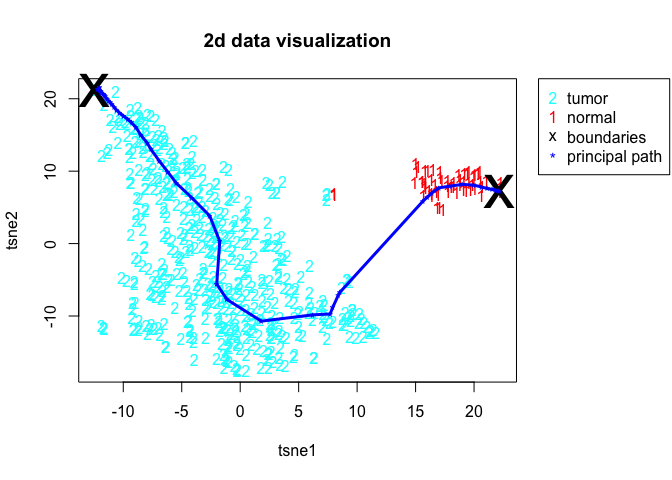Since in this example we have a n-dimensional space (with n = 19637), a dimensionality reduction strategy is necessary to plot the principal path algorithm. spathial uses the t-SNE algorithm .

The spathial method spathialStatistics can be used to calculate the Pearson’s correlation coefficients, the p values, the adjusted p values and rank the genes according to the correlation scores:

spathial_statistics <- spathial::spathialStatistics(spathial_res)

In this way it is possible to find the genes involved in the transition from “Normal” to “Tumor” (genes with high correlation coeffients and significant p values).

# An example with Single-cell RNA-Seq data

In this section a real case study is shown on the Karlsson Single-cell RNA-Seq dataset . In this case the input matrix X is composed by 23928 features (genes) and 96 samples. Each column of matrix X represents a feature and each row is the gene expression profile of a specific cell. The vector X_labels contains the annotations of the cell (“G1”, “S” or “G2/M”) which describes the phase of the cell cycle. We provide an .rda file with both the X and X_labels. Users can simply load it by computing the following commands.

load(url("https://github.com/erikagardini/spathial_dataframes/raw/master/karlsson-rawcounts.rda"))

#NORMALIZATION AND PREFILTERING OF THE DATA (GENES IN AT LEAST 3 CELLS)
#library(Seurat)
#seuset<-CreateSeuratObject(counts=rawcounts,min.cells=3,min.features=200)
#normalized_seuset<-NormalizeData(seuset,normalization.method="LogNormalize",scale.factor=10000)
#normalized_expmat <- as.matrix(normalized_seuset[["RNA"]]@data)
#save(normalized_expmat,annotation,file="karlsson-normalized_expmat.rda")

X <- t(normalized_expmat)
X_labels <- annotation

The aim of the example is to navigate the space from the “G1” phase to the “G2/M” phase in order to find the genes involved in the cell cycle.

In this case, the start point is the centroid of the “G1” cells and the end point is the centroid of the “G2” cells. This is an existing spathialBoundaries mode, therefore the function spathialBoundaries can be used with mode=2 and the parameters from and to respectively equal to “G1” and “G2”.

The following code block shows how to set the boundaries:

boundaries <- spathial::spathialBoundaryIds(X, X_labels, mode=2, from="G1", to="G2/M")
boundary_ids<-boundaries$boundary_ids X <- boundaries$X
X_labels <- boundaries\$X_labels

In this case the prefiltering won’t be executed since one is searching for a global solution. The next step consists in running the principal path algorithm using the spathial method spathialWay. A path with 50 intermediate points (waypoints) can be obtained with NC=50.

spathial_res <- spathial::spathialWay(X, boundary_ids, NC = 50)

Each waypoint is a new cell which is sligthly different from the previous one and all of them are topologically connected via a chain of springs.

Finally, one can use the spathial method spathialPlot to plot the path as it follows:

spathial::spathialPlot(X, X_labels, boundary_ids, spathial_res, perplexity_value = 30, mask = NULL)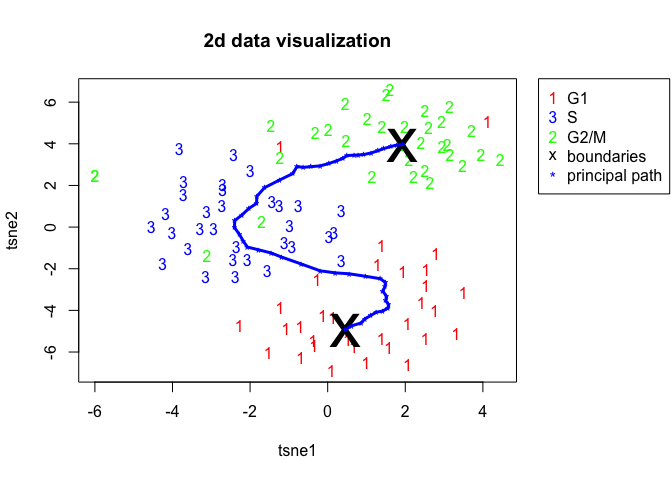Since in this example we have a n-dimensional space (with n = 23928), a dimensionality reduction strategy is necessary to plot the principal path. spathial uses the t-SNE algorithm .

The spathial method spathialStatistics can be used to calculate the Pearson’s correlation coefficients, the p values, the adjusted p values and rank the genes according to the correlation scores:

spathial_statistics <- spathial::spathialStatistics(spathial_res)

In this way it is possible to find the genes involved in the transition from “G1” to “G2/M” (genes with high correlation coeffients and significant p values).